Games
Problems
Go Pro!

# Featured Pro Problems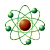A velociraptor falls from a cliff while a mosasaurus launches out of the water. Mayhem ensues.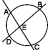Complex numbers, sequences and series, trigonometry, coordinate geometry, and conics all come into play in this hodge-podge problem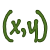Find the sum of x and y, if the following quadratic equations in x and y are true.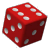Calculate the sum of squares, given the arithmetic, geometric and harmonic means of three numbersThree digits get together for brunch and discuss their relationships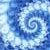Without a calculator find a nine digit number that meets the criteria of the problemA math problem involving Shrek wanting Fiona's telephone numberSolve the problem involving Luke Skywalker, Yoda, and an x-wing figher on Dagobah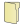Like us on Facebook to get updates about new resources